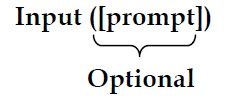#### Accepting input from Console

input() Function

Syntax for input() is:If prompt is present, it is displayed on monitor, after which the user can provide data from keyboard. Input takes whatever is typed from the keyboard and evaluates it. As the input provided is evaluated, it expects valid python expression. If the input provided is not correct then either syntax error or exception is raised by python.

Example

``````x= input ("enter data:")
Enter data: 2+1/2.0
Will supply 2.5 to x``````

input ( ), is not so popular with python programmers as:

i) Exceptions are raised for non-well formed expressions.

ii) Sometimes well formed expression can wreak havoc.

Output is what program produces. In algorithm, it was represented by print. For output in Python we use print. We have already seen its usage in previous examples. Let's learn more about it.

Example 1:input( ) with prompt string

``````>>> city=input (“Enter Your City: ”)
>>> print (“I am from “, city)

Example 2:input( ) without prompt string

``````>>> city=input()
Rajarajan
>>> print (I am from", city)
I am from Rajarajan``````

Example Program:

Python program to Input 2 Number and print its sum

``````x = int (input(“Enter Number 1: ”))
y = int (input(“Enter Number 2: ”))
print (“The sum = ”, x+y)``````

Output:

Enter Number 1: 34 Enter Number 2: 56 The sum = 90

Example 4: Alternate method for the above program

``````x,y=int (input("Enter Number 1 :")),int(input("Enter Number 2:"))
print ("X = ",x," Y = ",y)``````

Output:

Enter Number 1 :30 Enter Number 2:50 X = 30 Y = 50